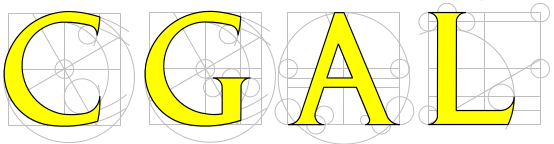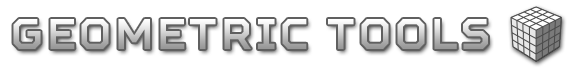### Introduction

The MicroStationAPI provides a C++ API for MicroStation developers wanting to create custom applications for MicroStation® from Bentley Systems.

MicroStation is a 3D computer-aided-design (CAD) tool and platform. Developers often want to write applications that manipulate 3D objects. Third-party computational geometry libraries may provide assistance.

### Third-Party Computational Geometry Libraries

There is a number of C++ third-party libraries that deal with computation geometry and related topics. Use one of these in your native-code application DLL built using the MicroStationAPI.

#### EIGENEIGEN is a C++ template library for linear algebra: matrices, vectors, numerical solvers, and related algorithms.

#### The Computational Geometry Algorithms LibraryThe Computational Geometry Algorithms Library (CGAL).

#### Geometric Tools#### The Matrix Template LibraryThe Matrix Template Library (MTL).

#### Boost.Geometry

The Boost.Geometry library provides geometric algorithms, primitives and spatial index.

#### Boost QVM

Boost quaternions, vectors and matrices (QVM) is a generic library for working with quaternions, vectors and matrices of static size with the emphasis on 2, 3 and 4-dimensional operations needed in graphics, video games and simulation applications.